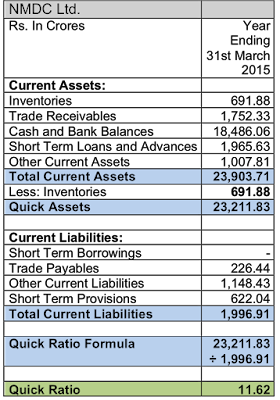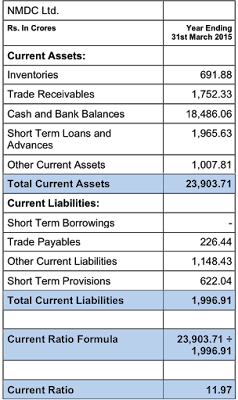## Friday, September 23, 2016

### Total Outside Liabilities to Tangible Net Worth (TOL/ TNW) Formula

#### TOL / TNW Formula:TOL/ TNW Ratio Formula

#### Meaning:

This ratio measures the total leverage employed by the business; meaning that the firm has used its net worth as a lever to raise outside funds.

Too much of leverage is not good as it may pose a problem for the organisation to repay the obligations during periods of stress.

#### Example:TOL/ TNW Example of NMDC Ltd.

#### Recommended Number:

TOL/ TNW shall be less than 3 - meaning that a business can have total outside liabilities of a maximum of three times its tangible net worth. Lower number means more safety and a higher number means weakness.

#### Infographic:

Created using Visme. An easy-to-use Infographic Maker.

### Debt Equity Ratio - Formula

#### Debt Equity Ratio Formula:Debt Equity Ratio Formula

#### Meaning:

Debt Equity Ratio measures the firm's ability to meet its long-term obligations on time, by comparing with the firm's own money or net worth. As a measure of additional safety, value of intangible assets like goodwill and brands are deducted from the net worth.

#### Example:Example Calculation of Debt Equity Ratio of NMDC Ltd.

#### Required Number:

The Debt Equity Ratio must be one or below. Lower the number than one so much stronger the company is and vise-versa.

### Quick Ratio - Formula

#### Quick Ratio Formula:Formula for Quick Ratio

#### Meaning:

Quick Ratio is the second important liquidity ratio that measures the organisation’s ability to meet immediate obligations on a much stringent method. Here inventories which are slower to realise or convert into cash are eliminated from the current assets.

#### Example:Example-Calculation of Quick Ratio of NMDC Ltd.

#### Desired Number:

The minimum required number for safety is 1. Below one means inadequate safety and any number more than one indicates increased margin of safety.

### Current Ratio - Formula

#### Current Ratio Formula:Formula for Calculation of Current Ratio

#### Meaning:

Current Ratio is the ratio or proportion between current assets and current liabilities. Current assets mean assets that can be expected to get converted into cash within a year and current liabilities are those that mature within 12 months. It is a measure of the business’s liquidity or the ability to meet short-term obligations.

#### Desirable Number:

Current ratio of two is minimum stipulated. Any number above means additional safety. A number below 2 indicates weakness.

Example:
Here we present the example of calculating the current ratio of NMDC Ltd., as per the balance sheet on 31st March 2015.Example Showing Current Ratio Calculation of NMDC Ltd.

### Earnings Per Share (EPS) - Formula

#### EPS Formula:EPS Formula

#### Definition:

Earnings Per Share (EPS) means the 'Net Profit' or 'Profit After Tax (PAT)' of a company reduced or converted to a single share.

#### Significance:

EPS is the important, denominator component in calculating Price to Earnings Ratio.

#### Example:

Let us consider the example of the EPS of SJVN Ltd., a company listed on Indian stock exchanges, for the financial year ending on 31st March 2015.Example Calculation of EPS of SJVN Ltd.